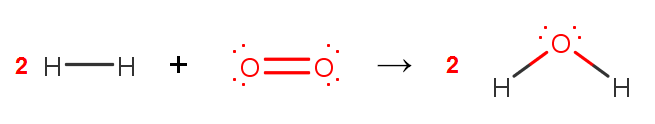# Problem: Consider the reaction: 2 H2(g) + O2(g) → 2 H2O(l). Use the bond enthalpies in the table to estimate ΔH for this reaction, ignoring the fact that water is in the liquid state.Average Bond Enthalpies (kJ/mol)C-H 413N-H 391O-H 463F-F 155C-C 348N-N 163O-O 146C=C 614N-O 201O=O 495Cl-F 253C-N 293N-F 272O-F 190Cl-Cl 242C-O 358N-Cl 200O-Cl 203Br-F 237C=O 799N-Br 243O-I 234Br-Cl 218C-F 485H-H 436Br-Br 193C-Cl 328H-F 567C-Br 276H-Cl 431I-Cl 208C-I 240H-Br 366I-Br 175H-I 299I-I 151

###### FREE Expert Solution

To calculate the ΔH°rxn using bond energies, we’re going to use the following equation:

2 H2(g) + O2(g) → 2 H2O(l)Calculate ΔH°rxn:

97% (384 ratings)###### Problem Details

Consider the reaction: 2 H2(g) + O2(g) → 2 H2O(l). Use the bond enthalpies in the table to estimate ΔH for this reaction, ignoring the fact that water is in the liquid state.

 Average Bond Enthalpies (kJ/mol) C-H 413 N-H 391 O-H 463 F-F 155 C-C 348 N-N 163 O-O 146 C=C 614 N-O 201 O=O 495 Cl-F 253 C-N 293 N-F 272 O-F 190 Cl-Cl 242 C-O 358 N-Cl 200 O-Cl 203 Br-F 237 C=O 799 N-Br 243 O-I 234 Br-Cl 218 C-F 485 H-H 436 Br-Br 193 C-Cl 328 H-F 567 C-Br 276 H-Cl 431 I-Cl 208 C-I 240 H-Br 366 I-Br 175 H-I 299 I-I 151

Frequently Asked Questions

What scientific concept do you need to know in order to solve this problem?

Our tutors have indicated that to solve this problem you will need to apply the Bond Energy concept. You can view video lessons to learn Bond Energy. Or if you need more Bond Energy practice, you can also practice Bond Energy practice problems.

What professor is this problem relevant for?

Based on our data, we think this problem is relevant for Professor Chatenever's class at University of Maine.